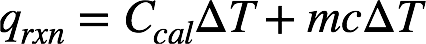# Problem: A 0.3423 g sample of pentane, C5H12, was burned in a bomb calorimeter. The temperature of the calorimeter and the 1.000 kg of water contained therein rose from 20.22 °C to 22.82 °C. The heat capacity of the calorimeter is 2.21 kJ/°C. The heat capacity of water = 4.184 J/g•°C. How much heat was given off during combustion of the sample of pentane?1) 8.8 kJ2) -8.8 kJ3) 16.6 J4) 16.6 kJ5) 3.1415 kJ

###### FREE Expert Solution
91% (261 ratings)
###### FREE Expert Solution

We’re being asked to calculate the heat of the reaction (qrxn) in a bomb calorimeter. This is given by the equation:where qcalorimeter = heat absorbed by the calorimeter and qsolution = heat absorbed by the water in the calorimeter.

Expanding this, we have:where

Ccal = heat capacity of the calorimeter

m = mass of water (in grams)

c = specific heat capacity of water

ΔT = change in temperature = final T – initial T.

We can’t use the mass of pentane to determine the heat of the reaction needed since the heat capacity of pentane isn’t given. This is why we used the heat absorbed by the calorimeter and the water instead.

91% (261 ratings)###### Problem Details

A 0.3423 g sample of pentane, C5H12, was burned in a bomb calorimeter. The temperature of the calorimeter and the 1.000 kg of water contained therein rose from 20.22 °C to 22.82 °C. The heat capacity of the calorimeter is 2.21 kJ/°C. The heat capacity of water = 4.184 J/g•°C. How much heat was given off during combustion of the sample of pentane?

1) 8.8 kJ

2) -8.8 kJ

3) 16.6 J

4) 16.6 kJ

5) 3.1415 kJ

What scientific concept do you need to know in order to solve this problem?

Our tutors have indicated that to solve this problem you will need to apply the Calorimetry concept. You can view video lessons to learn Calorimetry. Or if you need more Calorimetry practice, you can also practice Calorimetry practice problems.

What is the difficulty of this problem?

Our tutors rated the difficulty ofA 0.3423 g sample of pentane, C5H12, was burned in a bomb ca...as medium difficulty.

How long does this problem take to solve?

Our expert Chemistry tutor, Jules took 3 minutes and 27 seconds to solve this problem. You can follow their steps in the video explanation above.

What professor is this problem relevant for?

Based on our data, we think this problem is relevant for Professor Castaneda's class at UMICH.